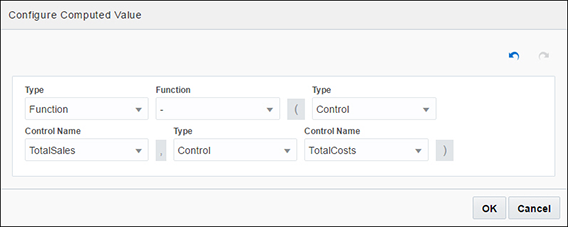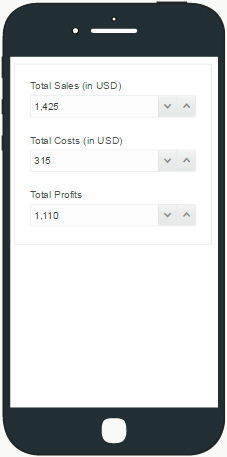## Create Computed Controls

Within a web form, you can define a control as a computed field that uses a data definition, value of an existing control, result of a custom formula, or connector data as its value.

You can define complex formulas using different functions based on data definitions, connector data, and existing controls to compute the control value. All controls that support binding also support computed values.

To convert an editable control to a computed control in a web form:

1. Click on the control in the form’s canvas and select the Computed Value check box on the Properties pane's General tab. An Edit button appears.

2. Click Edit to define how the control’s value is calculated.

3. In the Configure Computed Value window, select from the following options in the Type field:

1. Constant lets you specify a value for the selected control. Be sure to specify the corresponding value in the Value field.

2. Data Definition lets you use the data from the payload. When you click the adjacent Value field that displays, available items are listed.

3. Control lets you use the data from a control. Be sure to select the control name.

4. Function lets you define custom formulas using common operations with strings, values and arrays, as described in Specifying Functions.

5. Connector Data lets you assign a value from a global connector call.

4. After configuring the value, click OK, then Save.

Note:

• If a control has a computed value and also has a value assigned through payload, the computed value has the priority.

• If a control has a computed value but is edited, either through user input or event actions, the new value remains. However, when a dependency (data definition, control value, or connector data) is updated, the new computed value is set.

Example of a Computed Control

Let’s create a web form to collect sales and costs data through user input and calculate total profits. To create a web form from scratch, see Creating Web Forms.

When the form loads, you should be able to enter sales and costs data in editable controls, and a computed control should calculate the difference between data entered to arrive at total profits.

Now, let’s add and configure controls in the web form to suit this example.

1. Add and configure two editable number fields onto the form’s canvas.

1. From the Basic Palette, drag and drop a Number control onto the canvas.

2. Drag and drop another Number control below the first control.

3. Select the first Number control. On the General tab, change the Name field to TotalSales, and the Label field to Total Sales (in USD).

4. Repeat the previous step to change the second control’s name to TotalCosts and its label to Total Costs (in USD).

2. Add a third number field and configure it as a computed control.

1. Drag and drop a Number control below the previously added controls.

2. Select the control. On the General tab, change the Name field to TotalProfits, and the Label field to Total Profits. Select the Computed Value check box and click the Edit button.

3. In the Configure Computed Value window, define the formula to calculate profits.

Select Function in the Type field, and choose the Subtract (-) function in the Function field.

As illustrated in the following figure, specify parameters for additional fields to use values from editable controls.Description of the illustration configure-computed-control.png

Click OK to close the window.

3. Click Preview to test the computed control. The following figure shows sample data entered and profit calculated automatically.Description of the illustration computed-control-example.png